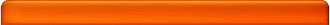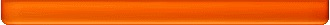# 4.0 GPA Scale Calculator

Many schools report your GPA on a 4.0 scale. Obtaining this grade at the end of the semester would be the equivalent of getting all A's or perfect scores in all your classes. If you have perfect scores all around then there is no need to use this calculator as your GPA is obviously 4.0. For the rest of us mortals, determining our grades is simple with this tool.

The "4.0" in the 4.0 GPA scale is identifying the highest possible grade that can be achieved for a course. In our calculator an A is equal to 4.0. If you needed to determine your grade on say a 5.0 GPA scale then an A or a perfect score would be 5.0 for a particular course.

The lowest possible grade is usually equal to a 0.0 on any scale and most times correlates to an "F" or the failing grade for the course. The scale coverts linearly from the 0.0 to 4.0 with your grade whether you are on a number or letter grading system for the class. The GPA scale conversion is usually given in the class syllabus.

A simplified 4.0 GPA scale:

4.0 = A = 90
3.0 = B = 80
2.0 = C = 70
1.0 = D = 60
0.0 = F = 50

The syllabus for the class will include more details such as conversions for B+ or 85% which could be equal to 3.5. Our calculator has a general 4.0 GPA scale that should work for most people.

## Use this calculator to determine your grade on a 4.0 GPA scale.

Instructions: Enter the Letter Grade and the Course Units (credits or hours)GPA: LetterGrade CourseUnits QualityPoints Course 1: A B C D F A- B+ B- C+ C- D+ D- x 0 1 2 3 4 5 6 7 8 9 10 = Course 2: A B C D F A- B+ B- C+ C- D+ D- x 0 1 2 3 4 5 6 7 8 9 10 = Course 3: A B C D F A- B+ B- C+ C- D+ D- x 0 1 2 3 4 5 6 7 8 9 10 = Course 4: A B C D F A- B+ B- C+ C- D+ D- x 0 1 2 3 4 5 6 7 8 9 10 = Course 5: A B C D F A- B+ B- C+ C- D+ D- x 0 1 2 3 4 5 6 7 8 9 10 = Course 6: A B C D F A- B+ B- C+ C- D+ D- x 0 1 2 3 4 5 6 7 8 9 10 = Course 7: A B C D F A- B+ B- C+ C- D+ D- x 0 1 2 3 4 5 6 7 8 9 10 = Course 8: A B C D F A- B+ B- C+ C- D+ D- x 0 1 2 3 4 5 6 7 8 9 10 =Grade Equivalence A 4.00 A- 3.67 B+ 3.33 B 3.00 B- 2.67 C+ 2.33 C 2.00 C- 1.67 D+ 1.33 D 1.00 D- 0.67 F 0.00